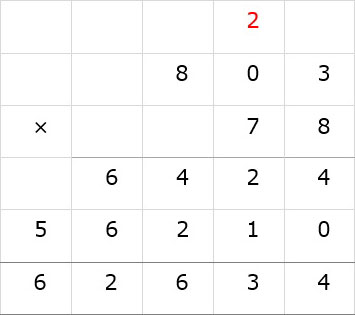# Multiplication of Large Numbers

Multiply 803 × 78

### Solution

Step 1:

First we multiply 8 of 78 with 3 of 803. 8 × 3 = 24. We bring down 4 and carry 2 to tens column.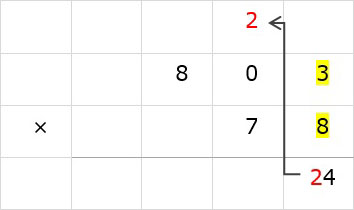Step 2:

Next we multiply 8 of 78 with 0 of 803. 8 × 0 = 0. 0 + 2 = 2. We bring down 2.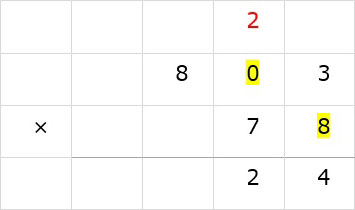Step 3:

Next we multiply 8 with 8 of 803. 8 × 8 = 64. We bring down 64 as there is nothing to carry to.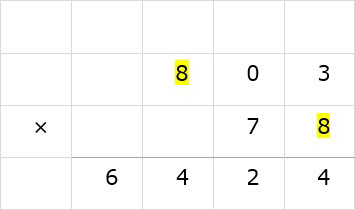Step 4:

Since multiplication in ones column is done we bring down a zero in next row in ones column. Next we multiply 7 of 78 with 3 of 803. 7 × 3 = 21. We bring down 1 and carry 2 to tens column.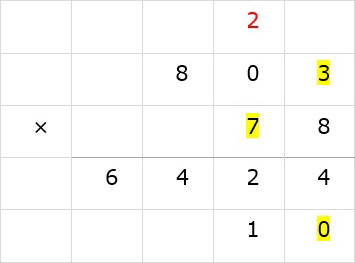Step 5:

Then we multiply 7 of 78 with 0 of 803. 7 × 0 = 0. 0 + 2 = 2. We bring down 2.Step 6:

Now we multiply 7 of 78 with 8 of 803. 7 × 8 = 56. We bring down 56 as there is nothing to carry to.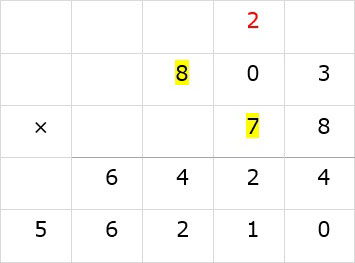Step 7: